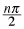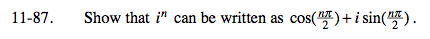Home > PC > Chapter 11 > Lesson 11.2.2 > Problem11-87

11-87.

Show that in can be written as cos() + i sin(). Homework Help ✎Recall from problem 11-85 that i can be written as:

Now use DeMoivre's theorem to write in.

$\textit{i}=\textit{z}=1\left( \cos\frac{\pi}{2}+\textit{i}\sin\frac{\pi}{2} \right)$